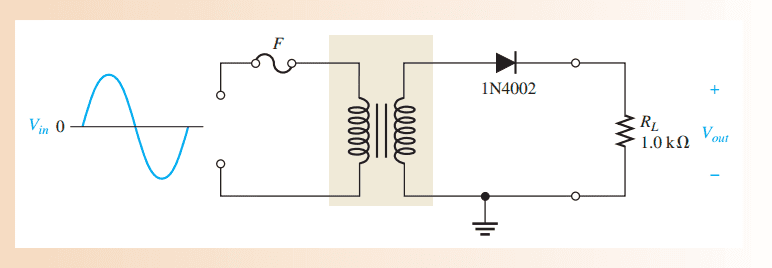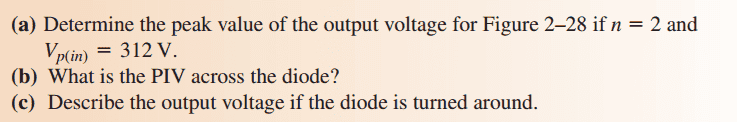# A problem in Coupling Transformers

Hello Guys !!

While Studing coupling transformers i found this problem
i tried to solve it
but i don't know if my solving is right or no !!
so tell me :)

The problem :My solve is :
V(out)=nV(in)
V(out)= 2 * 312 = 624 v
PIV = V(out) = 624 v
and if the diode is turned around it will be reversed biased so no current will pass across RL and
Output voltage will equal to zero ..

am i right guys or what ??

Thank you !!

gneill
Mentor
My solve is :
V(out)=nV(in)
V(out)= 2 * 312 = 624 v
PIV = V(out) = 624 v
Looks okay. Although whoever set the problem doesn't know his components very well. A 1N4002 diode as a PIV rating of about 100 V if I recall correctly. Expect the magic smoke to escape from the diode very shortly after switching on!

Also, you have ignored the ~0.7 V forward drop across the diode. Probably okay considering what a small percentage this is of the total voltage.

and if the diode is turned around it will be reversed biased so no current will pass across RL and
Output voltage will equal to zero ..
Nope. The secondary will present a full AC voltage signal, so if you turn the diode around it will simply conduct when the diode is forward biased on the opposite half-cycles (opposite that is to the half-cycles when it conducted in the original diode orientation). What does that tell you about the resulting potential across the load resistor?

What does that tell you about the resulting potential across the load resistor?

I think the potential across the load resistor will be the same but with an opposite sign
i mean that the voltage will take this shape ..am i right ??gneill
Mentor
I think the potential across the load resistor will be the same but with an opposite sign
i mean that the voltage will take this shape ..am i right ??Looks good!

•1 person